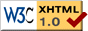# Draft Proposal for UCS-∞ Specification

Introduction | Properties | Details | Examples | Discussion | UCS-X Home Page

Authors: Tom Bishop (tbishop@wenlin.com) and Richard Cook (rscook@wenlin.com).

Updated October, 2009 (changes since 2007 are only stylistic).

Please Note: This is not a finalized specification. It is still at the "draft proposal" stage and may change.

## 1. Introduction

The name of this specification is UCS-∞.

• UCS = Universal (and Unlimited) Character Set (or Superset).
• ∞ = INFINITY sign (U+221E).

UCS-∞ enables unlimited extension of the Universal Character Set. It is a member of the UCS-X family of specifications. It specifies three encoding forms, UTF-∞-8, UTF-∞-16, and UTF-∞-32, which are compatible extensions of UTF-8, UTF-16, and UTF-32, respectively.

An infinite set of codes is defined. In practical implementations, maxima may be imposed by hardware limitations or other factors. Finite subsets of UCS-∞ can be defined (see conformance in the UCS-X specification).

## 2. Properties

UCS-∞ preserves and extends all the useful properties of UCS-G and UCS-E, with one qualification. In the finite encodings, the leading code unit indicates the length of a code. Since a single fixed-sized unit can't indicate the unlimited variable lengths of UCS-∞, this property requires modification. UCS-∞ does the next best thing by specifying the length in the first "few" units of a code, so that it is not necessary to scan all the way to the end of a code to determine its length.

## 3. Details

The set of UCS-∞ code points is the set of nonnegative integers. The notation for a code point is U+x, where x is any nonnegative hexadecimal integer. (Leading zeros are used if and only if there would otherwise be less than four digits.) As in Unicode, U+D800..U+DFFF ("surrogate code points") are excluded from the set of USV (UCS-∞ scalar values).

UCS-∞ specifies three encoding forms (using 8-bit, 16-bit, and 32-bit units) that each associate a unique code with each USV. Detailed specifications are provided for the three encoding forms. Please see:

## 4. Examples

```USV = U+0041 (the letter 'A')
UTF-∞-8  = 41
UTF-∞-16 = 0041
UTF-∞-32 = 00000041

USV = U+5B57 (the Chinese/Japanese/Korean character '字')
UTF-∞-16 = 5B57
UTF-∞-32 = 00005B57

USV = U+10FFFF (the last USV in the Unicode Standard)
UTF-∞-8  = F4 8F BF BF
UTF-∞-16 = DBFF DFFF
UTF-∞-32 = 0010FFFF

USV = U+110000 (the first USV beyond the U+10FFFF limit)
UTF-∞-8  = F4 90 80 80
UTF-∞-16 = DC04 DE80 DE00
UTF-∞-32 = 00110000

USV = U+7FFFFFFF (the last USV in the original ISO 10646 standard)
UTF-∞-8  = FD BF BF BF BF BF
UTF-∞-16 = DD0F DFFF DFFF DFFF
UTF-∞-32 = 7FFFFFFF

USV = U+80000000 (the first USV beyond the original ISO 10646)
UTF-∞-8  = FE 82 80 80 80 80 80
UTF-∞-16 = DD10 DE00 DE00 DE00
UTF-∞-32 = 80000000

USV = U+123456789
UTF-∞-8  = FE 84 A3 91 96 9E 89
UTF-∞-16 = DD24 DED1 DEB3 DF89
UTF-∞-32 = F0000012 E3456789

USV = U+123456789ABCDEF0123456789ABCDEF0
UTF-∞-8  = FF AE 80 92 8D 85 99 B8 A6 AB B3 9E
BC 81 88 B4 95 A7 A2 9A AF 8D BB B0
UTF-∞-16 = DDFF DE09 DE91 DF45 DECF DE26 DF5E DEDE
DFE0 DE48 DFA2 DF67 DF13 DEAF DE6F DEF0
UTF-∞-32 = FFAC1234 E56789AB ECDEF012 E3456789 EABCDEF0

USV = U+F...(ninety-eight F's omitted)...F
UTF-∞-8  = FF B4 A5 A2 80 8F BF ...(sixty-four BF's omitted) ... BF
UTF-∞-16 = DDFF DE4D DE0F DFFF... (forty-two DFFF's omitted) ... DFFF
UTF-∞-32 = FFBA50FF EFFFFFFF ... (twelve EFFFFFFF's omitted) ... EFFFFFFF```

More examples are shown in the individual specifications for UTF-∞-8, UTF-∞-16, and UTF-∞-32.

## 5. Discussion

For storage of large code points, UTF-∞-32 is slightly more compact than UTF-∞-8, which in turn is slightly more compact than UTF-∞-16, as shown by the following table. For very large code points, the efficiency is determined almost entirely by the percentage of bits in each USV-storage unit that are actually used for USV-storage, rather than for overhead. UTF-∞-8 has overhead of at least two bits in each byte. UTF-∞-16 has overhead of at least seven bits in each 16-bit code unit. UTF-∞-32 has overhead of at least four bits in each 32-bit code unit.

Encoding formLower limit of overheadUpper limit of efficiency
UTF-∞-82/8 = 25.00%6/8 = 75.00%
UTF-∞-167/16 = 43.75%9/16 = 56.25%
UTF-∞-324/32 = 12.50%28/32 = 87.50%

All three forms are nevertheless reasonably efficient, and the above figures refer only to very large code points. Most real-life texts can be expected to contain mostly small code points, with relatively few large code points. For example, a text that is mostly ASCII, if stored as UTF-∞-8, might be considered to have close to 7/8 = 87.50% efficiency (which by coincidence is the same as UTF-∞-32 for large code points). UTF-∞-8 has the advantages of ASCII-compatibility and compactness of storing strings that are mostly ASCII. UTF-∞-16 has the advantages of UTF-16 compatibility and compactness of storing strings that are mostly CJK.

For implementation, applications, references, etc., please see the UCS-X page.